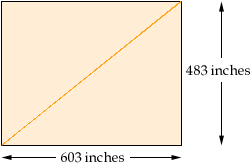From: Candace Education Level of question 8th grade Parent asking Building measuring 40 feet 3 inches by 50 feet 3inches What is the measurement of the diagonal of the building? What was method of obtainin answer? Hi Candace, I think that it is asked about the diagonal of the base of the building. So, now we have a rectangular base with length 40 feet 3 inches = 40*12 +3 inches = 483 inches and weight 50 feet 3 inches = 50*12 +3 inches = 603 inches.The triangle in the diagram is a right triangle. By the Pyphagorean Theorem the square on the diagonal is the sum of the squares on the other two sides. That is the square on the diaginal is 483*483 + 603*603 = 596898). The length of the diagonal is thus the square root of 596898 which is 772.6 inches. Cheers, Andrei Go to Math Central